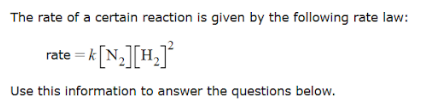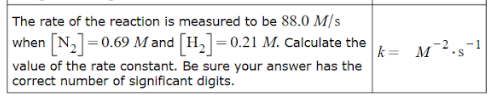# The rate of a certain reaction is given by the following rate law: rate = k[N2][H2]^2 Use this information to answer the questions below. The rate of the reaction is measured to be 88.0 M/s when [N2] = 0.69 M and [H2] = 0.21 M. Calculate the value of the rate constant. Be sure your answer has the correct number of significant digits.